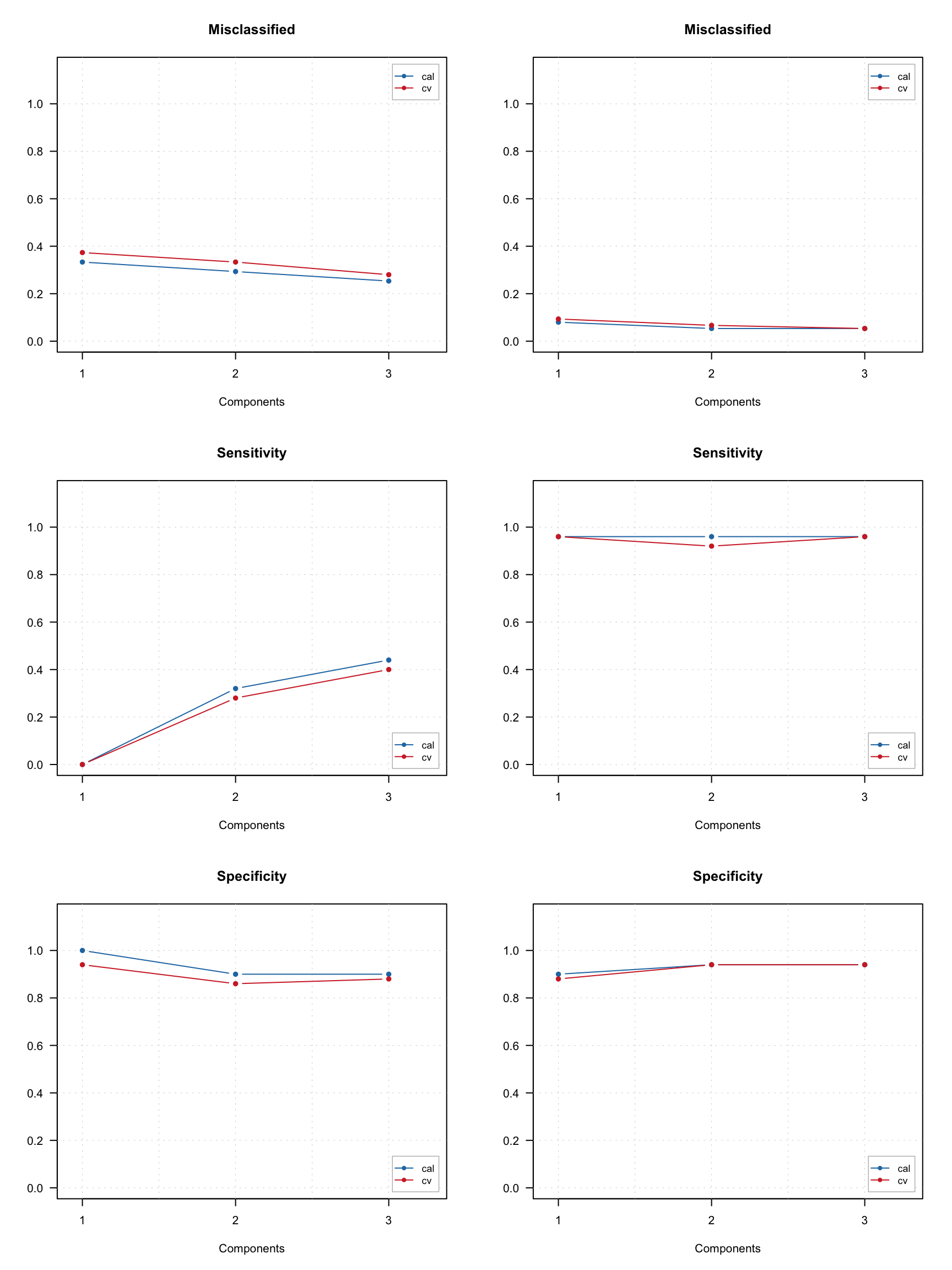## Randomization test

Another additional option for PLS regression implemented in mdatools is randomization test for estimation of optimal number of components. The description of the method can be found in this paper. The basic idea is that for each component from 1 to ncomp we compute a statistic $$T$$, which is a covariance between X-scores and the reference Y values. After that, this procedure is repeated for randomly permuted Y-values and distribution of the statistic is obtained. A parameter alpha is computed to show how often the statistic $$T$$, calculated for permuted Y-values, is the same or higher than the same statistic, calculated for original response values without permutations.

If a component is important, then the covariance for unpermuted data should be larger than the covariance for permuted data and therefore the value for alpha will be quite small (there is still a small chance to get similar covariance). This makes alpha very similar to p-value in a statistical test.

The function randtest() calculates alpha for each component, the values can be observed using summary() or plot() functions. There are also several functions, allowing e.g. to show distribution of statistics and the critical value for each component.

In example of code below most of the functions are shown.

data(people)

y = people[, 4, drop = FALSE]
X = people[, -4]

r = randtest(X, y, ncomp = 5, nperm = 1000, silent = TRUE)
summary(r)
##
## Summary for permutation test results
## Number of permutations: 1000
## Suggested number of components: 4
##
## Statistics and alpha values:
##              Comp 1    Comp 2    Comp 3    Comp 4     Comp 5
## Alpha     0.0520000 0.0000000 0.0000000 0.0000000 0.13200000
## Statistic 0.2403837 0.4349094 0.3571243 0.2678767 0.03603819

As you can see, alpha is very small for components 2–4 and then jumps up.

par( mfrow = c(2, 2))
plotHist(r, ncomp = 3)
plotHist(r, ncomp = 5)
plotCorr(r, ncomp = 3)
plotCorr(r, ncomp = 5)